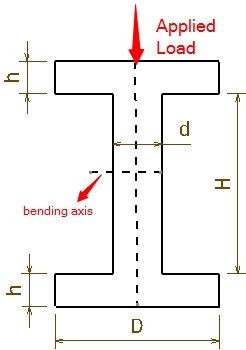Moment of Inertia Calculators - Different Shape Size Profiles
Hits: 1144
This is the I Beam Moment of Inertia Calculator. Simply enter the dimensions below for I beam to find the moment of inertia.d = h = D = H = h is the thickness of the web flange.d is the thickness of the center beam.H is the height of the center beam.D is the width of the web flange.